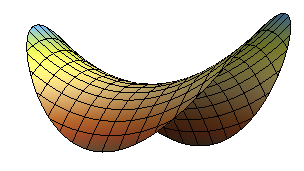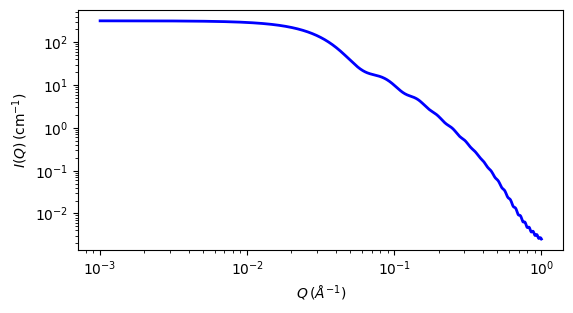# pringle¶

The Pringle model provides the form factor, $$P(q)$$, for a ‘pringle’ or ‘saddle-shaped’ disc that is bent in two directions.

Parameter

Description

Units

Default value

scale

Scale factor or Volume fraction

None

1

background

Source background

cm-1

0.001

60

thickness

Thickness of pringle

10

alpha

Curvature parameter alpha

None

0.001

beta

Curvature paramter beta

None

0.02

sld

Pringle sld

10-6-2

1

sld_solvent

Solvent sld

10-6-2

6.3

The returned value is scaled to units of cm-1 sr-1, absolute scale.

Definition

The form factor for this bent disc is essentially that of a hyperbolic paraboloid and calculated as

$P(q) = (\Delta \rho )^2 V \int^{\pi/2}_0 d\psi \sin{\psi} sinc^2 \left( \frac{qd\cos{\psi}}{2} \right) \left[ \left( S^2_0+C^2_0\right) + 2\sum_{n=1}^{\infty} \left( S^2_n+C^2_n\right) \right]$

where

$C_n = \frac{1}{r^2}\int^{R}_{0} r dr\cos(qr^2\alpha \cos{\psi}) J_n\left( qr^2\beta \cos{\psi}\right) J_{2n}\left( qr \sin{\psi}\right)$
$S_n = \frac{1}{r^2}\int^{R}_{0} r dr\sin(qr^2\alpha \cos{\psi}) J_n\left( qr^2\beta \cos{\psi}\right) J_{2n}\left( qr \sin{\psi}\right)$

and $$\Delta\rho\text{ is }\rho_{pringle}-\rho_{solvent}$$, $$V$$ is the volume of the disc, $$\psi$$ is the angle between the normal to the disc and the q vector, $$d$$ and $$R$$ are the “pringle” thickness and radius respectively, $$\alpha$$ and $$\beta$$ are the two curvature parameters, and $$J_n$$ is the nth order Bessel function of the first kind.Fig. 34 Schematic of model shape (Graphic from Matt Henderson, matt@matthen.com)¶Fig. 35 1D plot corresponding to the default parameters of the model.¶

Source

pringle.py $$\ \star\$$ pringle.c $$\ \star\$$ gauss76.c $$\ \star\$$ sas_JN.c $$\ \star\$$ sas_J1.c $$\ \star\$$ sas_J0.c $$\ \star\$$ polevl.c

Reference

1. Karen Edler, Universtiy of Bath, Private Communication. 2012. Derivation by Stefan Alexandru Rautu.

1. Onsager, Ann. New York Acad. Sci., 51 (1949) 627-659

Authorship and Verification

• Author: Andrew Jackson Date: 2008